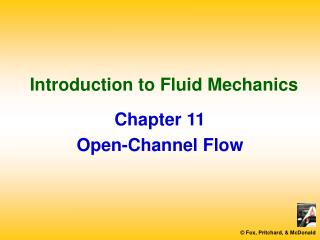# Introduction to Fluid Mechanics - PowerPoint PPT PresentationDownload PresentationIntroduction to Fluid Mechanics

Download Presentation## Introduction to Fluid Mechanics

- - - - - - - - - - - - - - - - - - - - - - - - - - - E N D - - - - - - - - - - - - - - - - - - - - - - - - - - -
##### Presentation Transcript

1. Introduction to Fluid Mechanics Chapter 11 Open-Channel Flow

2. Main Topics • Steady Uniform Flow • Specific Energy, Momentum Equation, and Specific Force • Steady, Gradually Varied Flow • Rapidly Varied Flow • Discharge Measurement

3. Steady Uniform Flow • Control Volume

4. Steady Uniform Flow • Chezy Equation • Chezy Coefficient

5. Steady Uniform Flow • Manning Equation (SI) • Manning Equation (US Customary)

6. Steady Uniform Flow • Manning Roughness Coefficients

7. Specific Energy, Momentum Equation, and Specific Force • Specific Energy

8. Specific Energy, Momentum Equation, and Specific Force • Froude Number Criterion • Froude Number Criterion (Rectangular Channel)

9. Specific Energy, Momentum Equation, and Specific Force • Momentum Equation

10. Specific Energy, Momentum Equation, and Specific Force • Momentum Equation (Steady State)

11. Specific Energy, Momentum Equation, and Specific Force • Specific Force

12. Specific Energy, Momentum Equation, and Specific Force • Critical Flow Examples